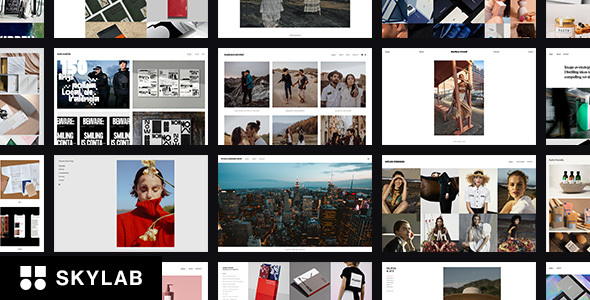4.5 Average out of 311 ratings\$59

## Theme Description

##### Build any layout you can imagine with intuitive drag and drop builder.## Change Log

```=== 3.9.2 (Jun 01, ‘23) ===
Plugins updated.
```
```=== 3.9.1 (Mar 12, ‘23) ===
Small bugs fixes.
Overall improvements.
```
```=== 3.9 (Oct 15, ‘22) ===
Plugins updated.
Overall improvements.
```
```=== 3.8.9 (Jul 06, ‘22) ===
Plugins updated.
Overall improvements.
```
```=== 3.8.8 (May 20, ‘22) ===
Plugins updated.
Overall improvements.
```
```=== 3.8.7 (Jan 08, ‘22) ===
Small bugs fixes.
```
```=== 3.8.6 (Jan 06, ‘22) ===
Plugins updated.
Overall improvements.
```
```=== 3.8.5 (Oct 31, ‘21) ===
Plugins updated.
Overall improvements.
```
```=== 3.8.4 (Aug 09, ‘21) ===
Plugins updated.
Overall improvements.
```
```=== 3.8.3 (Jun 12, ‘21) ===
Plugins updated.
Overall improvements.
```
```=== 3.8.1 (Mar 18, ‘21) ===
Plugins updated.
```
```=== 3.8 (Dec 23, ‘20) ===
Plugins updated.
```
```=== 3.7 (Dec 03, ‘20) ===
Overall improvements.
```
```=== 3.6 (Oct 12, ‘20) ===
Overall improvements.
```
```=== 3.5 (Sep 15, ‘20) ===
Overall improvements.
```
```=== 3.4 (Jun 18, ‘20) ===
Overall improvements.
```
```=== 3.3.2 (May 9, ‘20) ===
Overall improvements.
```
```=== 3.3 (Mar 7, ‘20) ===
Overall improvements.
```
```=== 3.2 (Dec 22, ‘19) ===
Small bugs fixes.
```
```=== 3.1 (Dec 19, ‘19) ===
Theme options updated.
Overall improvements.
```
```=== 3.0.6 (Jul 11, ‘19) ===
Small bugs fixes.
```
```=== 3.0.5 (Apr 16, ‘19) ===
Small bugs fixes.
```
```=== 3.0.4 (Feb 5, ‘19) ===
Small bugs fixes.
```
```=== 3.0.3 (Dec 26, ‘18) ===
Small CSS fixes.
```
```=== 3.0.2 (Dec 1, ‘18) ===
Small CSS fixes.
```
```=== 3.0.1 (Nov 3, ‘18) ===
Small CSS fixes.
```
```=== 3.0 (Oct 8, ‘18) ===
Overall improvements.
```
```=== 2.1.1 (Apr 27, ‘16) ===
Fixed: R Slider gallery.
```
```=== 2.1.0 (Apr 20, ‘16) ===
```
```=== 2.0.9 (Feb 1, ‘16) ===
```
```=== 2.0.8 (Jan 1, ‘16) ===
```
```=== 2.0.7 (Oct 6, ‘15) ===
```
```=== 2.0.6 (Jul 12, ‘15) ===
Fixed: transitions for Internet Explorer.
```
```=== 2.0.5 (May 8, ‘15) ===
```
```=== 2.0.4 (May 1, ‘15) ===
Fixed: site title issue.
```
```=== 2.0.3 (Apr 13, ‘15) ===
Fixed: Portfolio Post Type plugin issue.
```
```=== 2.0.2 (Apr 10, ‘15) ===
Fixed: Retina logo issue.
```
```=== 2.0.1 (Apr 4, ‘15) ===
```
```=== 2.0 (Jan 26, ‘15) ===
New version
```
```=== 1.2.4 (Dec 17, ‘14) ===
```
```=== 1.2.3 (Sep 8, ‘14) ===
```
```=== 1.2.2 (Jul 3, ‘14) ===
Fixed: shortcode button.
```
```=== 1.2.1 (Apr 26, ‘14) ===
Fixed: insert shortcode button bug.
```
```=== 1.2 (Feb 27, ‘14) ===
Fixed: slider in IE11 bug.
```
```=== 1.1.9 (Nov 28, ‘13) ===
```
```=== 1.1.8 (Nov 7, ‘13) ===
```
```=== 1.1.7 (Sep 4, ‘13) ===
Fixed: bug in Galleries List (Fixed Width) page template.
```
```=== 1.1.6 (Aug 21, ‘13) ===
Fixed: fullscreen and full width sliders on Internet Explorer 8 browser.
```
```=== 1.1.5 (Aug 20, ‘13) ===
Fixed: white logo in fullscreen gallery.
```
```=== 1.1.4 (Aug 19, ‘13) ===
Added: Galleries List (Fixed Width) page template.
Added: gallery title and meta position option.
```
```=== 1.1.3 (Aug 17, ‘13) ===
Fixed: filter function in galleries list.
```
```=== 1.1.2 (Aug 17, ‘13) ===
Fixed: Tagline CSS bug in full-screen gallery.
```
```=== 1.1.1 (Aug 16, ‘13) ===
Fixed: CSS bugs in custom styles.
```
```=== 1.1 (Aug 6, ‘13) ===
```
```=== 1.0.9 (Aug 3, ‘13) ===
Fixed: compatible with WordPress 3.6.
```
```=== 1.0.8 (Aug 1, ‘13) ===
Fixed: minor bugs.
```
```=== 1.0.7 (Jul 19, ‘13) ===
Fixed: column's width in portfolios list.
Added: an updated version of Slider Revolution Responsive WordPress Plugin
```
```=== 1.0.6 (Jul 16, ‘13) ===
Fixed: column's width in galleries list.
```
```=== 1.0.5 (Jul 14, ‘13) ===
Fixed: autoplay function in “Full Width of Window” page template.
```
```=== 1.0.4 (Jul 12, ‘13) ===
Fixed: bug in “Masonry (no margins)” page template.
```
```=== 1.0.3 (Jun 25, ‘13) ===
Fixed: custom URL for related projects.
```
```=== 1.0.2 (Jun 18, ‘13) ===
```=== 1.0.1 (Jun 10, ‘13) ===
```=== 1.0 (May 21, ‘13) ===